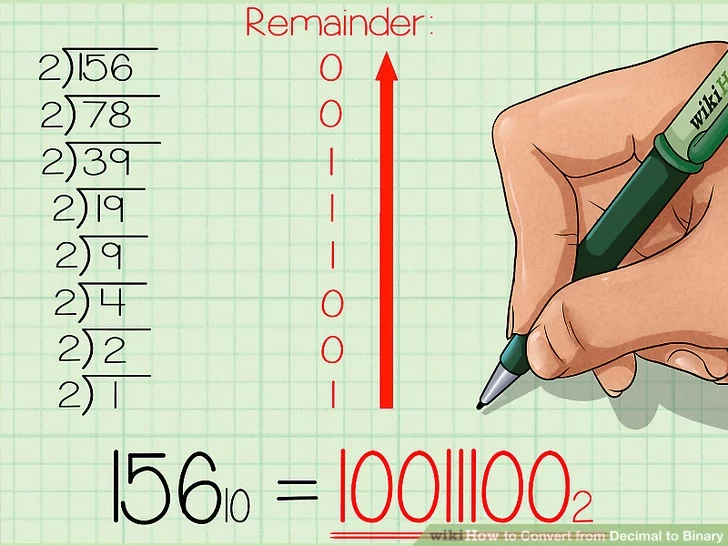# Number Systems

### Number Types

The number system holds significance in terms of writing and expressing code to a computer, typically in a programming language. Note that we (as humans) do not use hex or binary numbers that much outside of the computer science domain. For example, we don't walk into a supermarket and read prices in binary such as `\$0x10` :)

Often times in programming, we need to express numbers more quickly, and we might say int `x = 0x10000000` to quickly indicate 32-bit value with `bit31` set to 1. Notation `x = 0x10000000` is easier than writing `x = 268435456` which would be more cryptic for a programmer to realize the significance of because the reader of the programming code will not be able to quickly realize that it is specifically setting `bit31` to value of `1`.

#### Decimal

Typical numbers we are familiar with are decimals which are technically "base 10" numbers. So an ordinary number that we may be aware of such as 123 can be written as 12310.

The number 123 could also be written as:

`1*102 +2*101 +3*100` which is equal to `100 + 20 + 3 =12310`

#### Binary

Binary numbers are always 1s and 0s only. Similar to decimal numbers, binary numbers increase in powers of 2, rather than powers of 10. Binary numbers are written by with the "0b" notation, such as 0b1100

For example, binary `101` or `0b101` can be written as:

`1*22 +0*21 +1*20` which is equal to `4 + 0 + 1 = 510`

#### Hex

One digit of a hex number can count from 0-15, but since we have to represent the hex number using a single character, the numbers 0-9 are usual numbers, and the numbers 10-15 are represented by A, B, C, D, E, F

Where decimal is a power of 10, and binary is power of 2, hex numbers are powers of 16. Hex numbers are written with the "0x" notation, such as 0x10.

For example, hex `0x12` can be written as:

`1*161 +2*160` which is equal to `16 + 2 = 1810`

As another example, hex `0xC5` can be written as:

`12*161 +5*160` which is equal to `192 + 5 = 19710`

### Exercises

#### Decimal to Binary

Decimal (base 10) numbers can be converted in a couple of different ways as described here. One of the methods is to continue dividing by 2 and note down the remainder as described in the image below. The article above also describes a potentially faster method of conversion so be sure to read it!Please try converting the following to binary:

1. 125
2. 255
3. 500

#### Decimal to Hex

Decimal to hex is similar to Decimal to Binary except that we are dealing with powers of 16 rather than powers of 2.

My favorite method of conversion from decimal to hex is to first convert the number to binary. For example, let's start with a large number such as 23912. We can use the Decimal to Binary method to convert this first to binary:

• `2391210`
• `0b101110101101000`
• Split it up to nibbles:
• `0b101 1101 0110 1000`
• Then use the lookup table listed in Hex to Binary:
• `0x5D68`

Please try converting the following to hex:

1. 125
2. 255
3. 500

#### Hex to Binary

The following table can be utilized to convert hex to binary very instantly:

 x0 x1 x2 x3 x4 x5 x6 x7 x8 x9 xA xB xC xD xE xF 0000 0001 0010 0011 0100 0101 0110 0111 1000 1001 1010 1011 1100 1101 1110 1111

First row is HEX, and the second row is binary. For whatever hex number we wish to convert, we simply locate its equivalent in binary. For instance, if we wish to convert `0x5` to binary, it is `0b0101`, and `0xA5` would be `0b1010.0101` as you can convert one "nibble" (4-bits) at a time.

Let's take another example to convert `0x1BF` to binary; simply break it down by "nibbles":

• `0x1` --> `0b0001`
• `0xB` --> `0b1011`
• `0xF` --> `0x1111`
• Answer: `0b0001 1011 1111`

Please try converting the following to binary:

1. 0x55
2. 0x125
3. 0x40000000

#### Hex to Decimal

For Hex to Binary, we used a lookup table as a "cheat code" :). For Hex to decimal, it would be easier to re-write the numbers as powers of 16. For example, to convert `0x1BF` to decimal, we can break it down to:

• `0x1` --> `1 * 162` --> `256`
• `0xB` --> `11 * 161` --> `176`
• `0xF` --> `15 * 160` --> `15`
• `256+176+15 = 447`

Please try converting the following to decimal:

1. 0x55
2. 0x125
3. 0x40000000